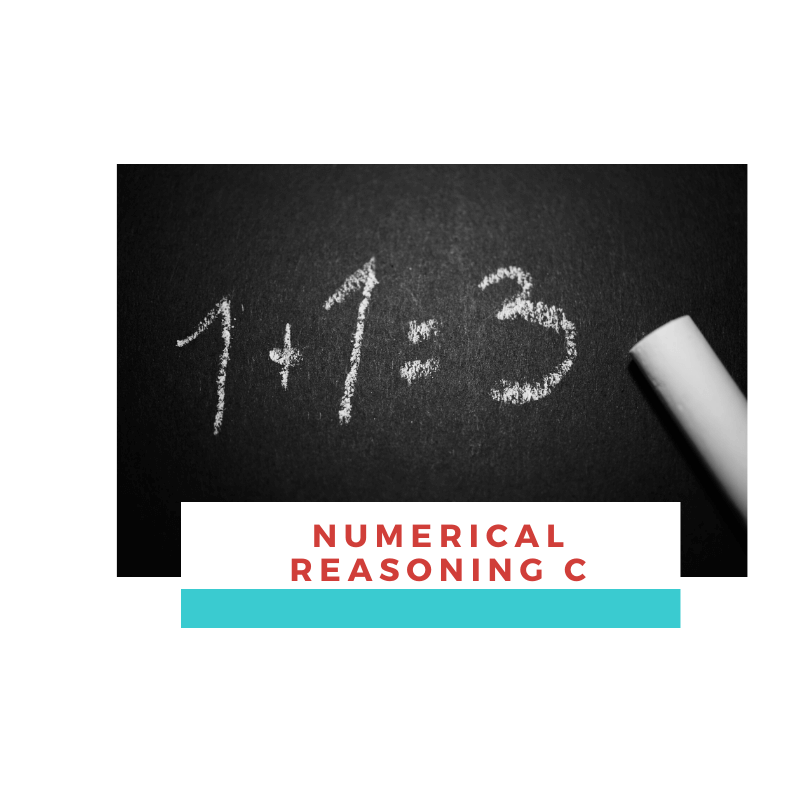## Numerical Reasoning C

##### Are you ready to take the upcoming Civil Service Exam?

Total items: 10 items
Duration: 10 minutes

Important: Prepare a pen and paper for math-related questions.

##### Topics
• Multiples and Factors
• Divisibility Rule
• Number Sequences
• PEMDAS
• Decimals
• Fractions
• Percent
• Ratio and Proportion
• Averages
• Word Problems

0%

You have 10 minutes to answer this quiz.Numerical Reasoning Part C

1 / 10

33/15 + 61/12 + 7/4 = ?

2 / 10

A store launched a promo of Buy 2 Take 1 for selected jackets.

Nora brought home three jackets from the sale. If she paid P920,

how much did she save?

3 / 10

17.92 + 20.15 + 0.99 =

4 / 10

The sum of a and b is 387, while c is equal to 117. What is the average of a, b and c?

5 / 10

What is the value of 17 – 3 (1 - 22 ) + 3- 8 x 2?

6 / 10

Find the value of X4 in the sequence given by Xn = 7 (2/7)n

7 / 10

13% of 258 is 78% of x. Find the value of x.

8 / 10

Using “Divisibility Rules”, which of the following is not divisible by 4?

9 / 10

The Greatest Common Factor (GCF) of 81 and 243 is:

10 / 10

Mae is travelling at 73 kph while John is travelling at 85 kph. Both left the same place at the same time but in opposite directions. In how many hours will the two cars be 711 kilometers apart?

0%

Did you like this quiz?

## Explore Other Quizzes

#### Need More Quizzes?

Done answering FREE quizzes? Avail up to 50% OFF.

Z

#### Free Quizzes

Study and practice now using our FREE Quizzes.